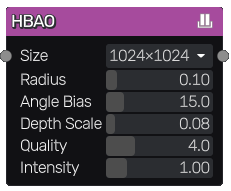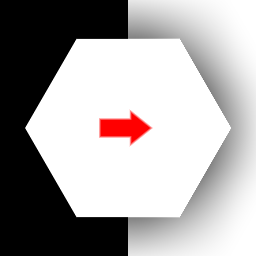# HBAO node¶

The HBAO node generates a horizon based ambient occlusion texture from its input.## Inputs¶

The HBAO node accepts a single greyscale image as input, interpreted as a heightmap.

## Outputs¶

The HBAO node outputs the generated oclusion map.

## Parameters¶

The HBAO node has the following parameters:

• the size of the ambient occlusion map

• the radius of affected range of point in UV size

• the angle bias - minimum angle on heightmap for AO to occur. Angle is calculated in UV space, where depth (grayscale input) is scaled by depth scale parameter

• the depth scale value controls cacluated normals & tangents by scaling range of values of grayscale input

• the quality controls number of steps per direction sampling

• the intensity of the generated ambient occlusion map

## Example images¶